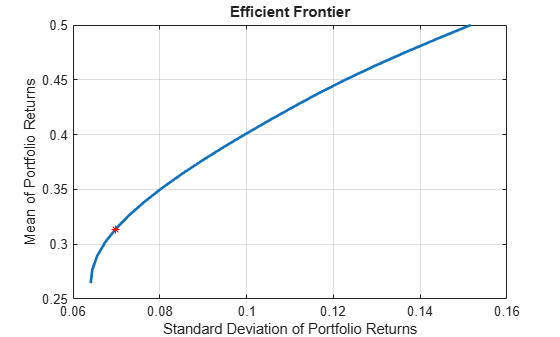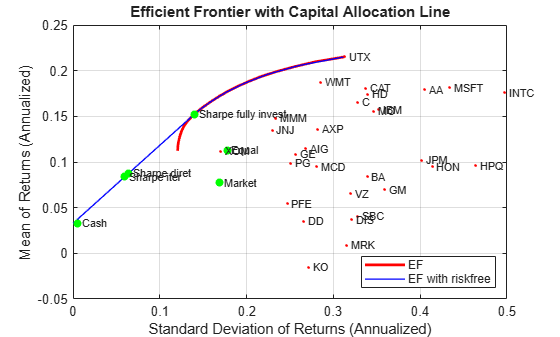# estimateMaxSharpeRatio

Estimate efficient portfolio to maximize Sharpe ratio for Portfolio object

## Syntax

``````[pwgt,pbuy,psell] = estimateMaxSharpeRatio(obj)``````
``````[pwgt,pbuy,psell] = estimateMaxSharpeRatio(___,Name,Value)``````

## Description

example

``````[pwgt,pbuy,psell] = estimateMaxSharpeRatio(obj)``` estimates efficient portfolio to maximize Sharpe ratio for `Portfolio` object. For details on the workflow, see Portfolio Object Workflow.```

example

``````[pwgt,pbuy,psell] = estimateMaxSharpeRatio(___,Name,Value)``` adds optional name-value pair arguments. ```

## Examples

collapse all

Estimate the efficient portfolio that maximizes the Sharpe ratio. The `estimateMaxSharpeRatio` function maximizes the Sharpe ratio among portfolios on the efficient frontier. This example uses the default `'direct'` method to estimate the maximum Sharpe ratio. For more information on the `'direct`' method, see Algorithms.

```p = Portfolio('AssetMean',[0.3, 0.1, 0.5], 'AssetCovar',... [0.01, -0.010, 0.004; -0.010, 0.040, -0.002; 0.004, -0.002, 0.023]); p = setDefaultConstraints(p); plotFrontier(p, 20); weights = estimateMaxSharpeRatio(p); [risk, ret] = estimatePortMoments(p, weights); hold on plot(risk,ret,'*r');```Estimate the efficient portfolio that maximizes the Sharpe ratio. The `estimateMaxSharpeRatio` function maximizes the Sharpe ratio among portfolios on the efficient frontier. This example uses the `'direct'` method for a `Portfolio` object (`p`) that does not specify a tracking error and only uses linear constraints. The `setSolver` function is used to control the `SolverType` and` SolverOptions`. In this case, the `SolverType `is `quadprog`. For more information on the `'direct'` method, see Algorithms.

```p = Portfolio('AssetMean',[0.3, 0.1, 0.5], 'AssetCovar',... [0.01, -0.010, 0.004; -0.010, 0.040, -0.002; 0.004, -0.002, 0.023]); p = setDefaultConstraints(p); plotFrontier(p, 20); p = setSolver(p,'quadprog','Display','off','ConstraintTolerance',1.0e-8,'OptimalityTolerance',1.0e-8,'StepTolerance',1.0e-8,'MaxIterations',10000); weights = estimateMaxSharpeRatio(p); [risk, ret] = estimatePortMoments(p, weights); hold on plot(risk,ret,'*r');```Estimate the efficient portfolio that maximizes the Sharpe ratio. The `estimateMaxSharpeRatio` function maximizes the Sharpe ratio among portfolios on the efficient frontier. This example uses the `'direct'` method for a `Portfolio` object (`p`) that specifies a tracking error uses nonlinear constraints. The `setSolver` function is used to control the `SolverType `and` SolverOptions`. In this case `fmincon` is the `SolverType`.

```p = Portfolio('AssetMean',[0.3, 0.1, 0.5], 'AssetCovar',... [0.01, -0.010, 0.004; -0.010, 0.040, -0.002; 0.004, -0.002, 0.023],'lb', 0,'budget', 1); plotFrontier(p, 20); p = setSolver(p, 'fmincon', 'Display', 'off', 'Algorithm', 'sqp', ... 'SpecifyObjectiveGradient', true, 'SpecifyConstraintGradient', true, ... 'ConstraintTolerance', 1.0e-8, 'OptimalityTolerance', 1.0e-8, 'StepTolerance', 1.0e-8); weights = estimateMaxSharpeRatio(p); te = 0.08; p = setTrackingError(p,te,weights); [risk, ret] = estimatePortMoments(p,weights); hold on plot(risk,ret,'*r');```The `estimateMaxSharpeRatio` function maximizes the Sharpe ratio among portfolios on the efficient frontier. In the case of Portfolio with a risk-free asset, there are multiple efficient portfolios that maximize the Sharpe ratio on the capital asset line. Because of the nature of `'direct'` and `'iterative'` methods, the portfolio weights (`pwgts`) output from each of these methods might be different, but the Sharpe ratio is the same. This example demonstrates the scenario where the `pwgts` are different and the Sharpe ratio is the same.

```load BlueChipStockMoments mret = MarketMean; mrsk = sqrt(MarketVar); cret = CashMean; crsk = sqrt(CashVar); p = Portfolio('AssetList', AssetList, 'RiskFreeRate', CashMean); p = setAssetMoments(p, AssetMean, AssetCovar); p = setInitPort(p, 1/p.NumAssets); [ersk, eret] = estimatePortMoments(p, p.InitPort); p = setDefaultConstraints(p); pwgt = estimateFrontier(p, 20); [prsk, pret] = estimatePortMoments(p, pwgt); pwgtshpr_fully = estimateMaxSharpeRatio(p,'Method','direct'); [riskshpr_fully, retshpr_fully] = estimatePortMoments(p,pwgtshpr_fully); q = setBudget(p, 0, 1); qwgt = estimateFrontier(q, 20); [qrsk, qret] = estimatePortMoments(q, qwgt);```

Plot the efficient frontier with a tangent line (`0` to `1` cash).

```pwgtshpr_direct = estimateMaxSharpeRatio(q,'Method','direct'); pwgtshpr_iter = estimateMaxSharpeRatio(q,'Method','iterative'); % Default for 'TolX' is 1e-8 [riskshpr_diret, retshpr_diret] = estimatePortMoments(q,pwgtshpr_direct); [riskshpr_iter, retshpr_iter] = estimatePortMoments(q,pwgtshpr_iter); clf; portfolioexamples_plot('Efficient Frontier with Capital Allocation Line', ... {'line', prsk, pret, {'EF'}, '-r', 2}, ... {'line', qrsk, qret, {'EF with riskfree'}, '-b', 1}, ... {'scatter', [mrsk, crsk, ersk, riskshpr_fully, riskshpr_diret, riskshpr_iter], ... [mret, cret, eret, retshpr_fully , retshpr_diret, retshpr_iter], {'Market', 'Cash', 'Equal','Sharpe fully invest', 'Sharpe diret','Sharpe iter'}}, ... {'scatter', sqrt(diag(p.AssetCovar)), p.AssetMean, p.AssetList, '.r'}); ```When a risk-free asset is not available to the portfolio, or in other words, the portfolio is fully invested, the efficient frontier is curved, corresponding to the red line in the above figure. Therefore, there is a unique (risk, return) point that maximizes the Sharpe ratio, which the `'iterative'` and `'direct'` methods will both find. If the portfolio is allowed to invest in a risk-free asset, part of the red efficient frontier line is replaced by the capital allocation line, resulting in the efficient frontier of a portfolio with a risk-free investment (blue line). All the (risk, return) points on the straight blue line share the same Sharpe ratio. Also, it is likely that the `'iterative'` and `'direct'` methods end up with different points, therefore there are different portfolio allocations.

When using the `'iterative'` method, you can use an optional `'TolX'` name-value argument. `TolX` is a termination tolerance that is related to the possible return levels of the efficient frontier. If the selected `TolX` value is large compared to the range of returns, then the accuracy of the solution is poor. `TolX` should be a number smaller than 0.01*(`maxReturn` - `minReturn`).

```maxReturn = max(qret); % Max return portfolio minReturn = min(qret); % Min return portfolio display(0.01*(maxReturn-minReturn))```
``` 1.5193e-04 ```

The purpose of increasing the termination tolerance is to speed up the convergence of the `'iterative'` algorithm. However, as previously mentioned, the accuracy of the solution will decrease. You can see this in the table that follows.

```pwgtshpr_iter_largerTol = estimateMaxSharpeRatio(q, 'Method', 'iterative',... 'TolX', 1e-4); display(table(pwgtshpr_iter, pwgtshpr_iter_largerTol,... 'VariableNames', {'Default TolX = 1e-8','TolX = 1e-4'}))```
``` 30x2 table Default TolX = 1e-8 TolX = 1e-4 ___________________ ___________ 0 0 0 0 0 0 0 0 0 0 0 0 0 0 0 0 0 0 0 0 0 0 0 0 0 0 0 0 0.009173 0.0091742 0.031008 0.031011 0 0 0 0 0 0 0.053382 0.053388 0.048198 0.048204 0 0 0.01542 0.015422 0 0 0.025685 0.025688 0 0 0.020681 0.020684 0 0 0.075935 0.075945 0.064881 0.064889 ```

In the table, the value of the weights varies slightly with respect to the weights obtained with the default tolerance, as expected.

Create a `Portfolio` object for three assets.

```AssetMean = [ 0.0101110; 0.0043532; 0.0137058 ]; AssetCovar = [ 0.00324625 0.00022983 0.00420395; 0.00022983 0.00049937 0.00019247; 0.00420395 0.00019247 0.00764097 ]; p = Portfolio('AssetMean', AssetMean, 'AssetCovar', AssetCovar); p = setDefaultConstraints(p); ```

Use `setBounds` with semicontinuous constraints to set xi = `0` or `0.02` <= `xi` <= `0.5` for all i = `1`,...`NumAssets`.

`p = setBounds(p, 0.02, 0.5,'BoundType', 'Conditional', 'NumAssets', 3); `

When working with a `Portfolio` object, the `setMinMaxNumAssets` function enables you to set up cardinality constraints for a long-only portfolio. This sets the cardinality constraints for the `Portfolio` object, where the total number of allocated assets satisfying the nonzero semi-continuous constraints are between `MinNumAssets` and `MaxNumAssets`. By setting `MinNumAssets` = `MaxNumAssets` = 2, only two of the three assets are invested in the portfolio.

`p = setMinMaxNumAssets(p, 2, 2); `

Use `estimateMaxSharpeRatio `to estimate efficient portfolio to maximize Sharpe ratio.

`weights = estimateMaxSharpeRatio(p,'Method','iterative')`
```weights = 3×1 0 0.5000 0.5000 ```

The `estimateMaxSharpeRatio` function uses the MINLP solver to solve this problem. Use the `setSolverMINLP` function to configure the `SolverType` and options.

`p.solverOptionsMINLP`
```ans = struct with fields: MaxIterations: 1000 AbsoluteGapTolerance: 1.0000e-07 RelativeGapTolerance: 1.0000e-05 NonlinearScalingFactor: 1000 ObjectiveScalingFactor: 1000 Display: 'off' CutGeneration: 'basic' MaxIterationsInactiveCut: 30 ActiveCutTolerance: 1.0000e-07 IntMainSolverOptions: [1x1 optim.options.Intlinprog] NumIterationsEarlyIntegerConvergence: 30 ExtendedFormulation: 0 NumInnerCuts: 10 NumInitialOuterCuts: 10 ```

## Input Arguments

collapse all

Object for portfolio, specified using a `Portfolio` object.

Note

The risk-free rate is obtained from the property `RiskFreeRate` in the Portfolio object. If you leave the `RiskFreeRate` unset, it is assumed to be `0`. If the max return of portfolio is less than the `RiskFreeRate`, the solution is set as `pwgt` at max return and the resulting Sharpe ratio will be negative.

Data Types: `object`

### Name-Value Arguments

Specify optional pairs of arguments as `Name1=Value1,...,NameN=ValueN`, where `Name` is the argument name and `Value` is the corresponding value. Name-value arguments must appear after other arguments, but the order of the pairs does not matter.

Before R2021a, use commas to separate each name and value, and enclose `Name` in quotes.

Example: ```[pwgt,pbuy,psell] = estimateMaxSharpeRatio(p,'Method’,'iterative')```

Method to estimate Sharpe ratio, specified as the comma-separated pair consisting of `'Method'` and a character vector with one of the following values:

Note

If you are using `estimateMaxSharpeRatio` with a `Portfolio` object with semicontinuous and cardinality constraints specified by `setBounds` and `setMinMaxNumAssets`, you can only use the `'iterative'` method.

Data Types: `char`

Since R2022a

Tolerance on the step size for `fminbnd` convergence when using `'iterative'` method, specified as the comma-separated pair consisting of `'TolX'` and a positive scalar. The step size is related to the return levels achieved on the efficient frontier and tried by `fminbnd`. If the specified `TolX` is large compared to the range of returns, the accuracy of the solution is poor. `TolX` should be a number smaller than 0.01*(`maxReturn` - `minReturn`).

Note

If the `'direct'` method is selected, `TolX` is ignored.

Data Types: `double`

## Output Arguments

collapse all

Portfolio on the efficient frontier with a maximum Sharpe ratio, returned as a `NumAssets` vector.

Purchases relative to an initial portfolio for a portfolio on the efficient frontier with a maximum Sharpe ratio, returned as a `NumAssets` vector.

`pbuy` is returned for a `Portfolio` input object (`obj`).

Sales relative to an initial portfolio for a portfolio on the efficient frontier with maximum Sharpe ratio, returned as a `NumAssets` vector.

`psell` is returned for a `Portfolio` input object (`obj`).

collapse all

### Sharpe Ratio

The Sharpe ratio is the ratio of the difference between the mean of portfolio returns and the risk-free rate divided by the standard deviation of portfolio returns.

The `estimateMaxSharpeRatio` function maximizes the Sharpe ratio among portfolios on the efficient frontier.

## Tips

You can also use dot notation to estimate an efficient portfolio that maximizes the Sharpe ratio.

`[pwgt,pbuy,psell] = obj.estimateMaxSharpeRatio;`

## Algorithms

The maximization of the Sharpe ratio is accomplished by either using the `'direct'` or `'iterative'` method. For the `'direct'` method, consider the following scenario. To maximize the Sharpe ratio is to:

where μ and C are the mean and covariance matrix, and rf is the risk-free rate.

If μT x - rf ≤ 0 for all x the portfolio that maximizes the Sharpe ratio is the one with maximum return.

If μT x - rf > 0, let $t=\frac{1}{{\mu }^{T}x-{r}_{f}}$

and y = tx (Cornuejols  section 8.2). Then after some substitutions, you can transform the original problem into the following form,

Only one optimization needs to be solved, hence the name “direct”. The portfolio weights can be recovered by x* = y* / t*.

For the `'iterative'` method, the idea is to iteratively explore the portfolios at different return levels on the efficient frontier and locate the one with maximum Sharpe ratio. Therefore, multiple optimization problems are solved during the process, instead of only one in the `'direct'` method. Consequently, the `'iterative'` method is slow compared to `'direct'` method.

 Cornuejols, G. and Reha Tütüncü. Optimization Methods in Finance. Cambridge University Press, 2007.